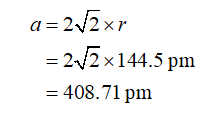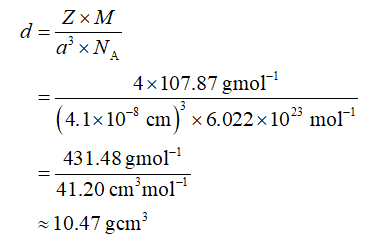# Crystallographic analysis of an unknown metal sample indicates that it crystallizes in a cubic close-packed arrangement with a radius of 144.5 pm. Based on the data tabulated below, what is the identity of the unknown?MetalDensity (g/cm3)Ag10.5Cu8.96Rh12.4Sr2.64

Question
1 views

Crystallographic analysis of an unknown metal sample indicates that it crystallizes in a cubic close-packed arrangement with a radius of 144.5 pm. Based on the data tabulated below, what is the identity of the unknown?

 Metal Density (g/cm3) Ag 10.5 Cu 8.96 Rh 12.4 Sr 2.64
check_circle

Step 1

The relation between the radius of the unit cell (r) and the edge length of the unit cell (a) is shown below. The value for r is substituted in the equation, shown below. The edge length for the cubic close-packed unit cell is 408.71 pm.Step 2

According to the unit conversion, 1pm is equal to 1×10-10cm thus 408.71 pm is approximately equal to 4.01×10-8 cm. If the silver element considered as the unknow element the density of unit cell for the cubic close pack is calculated as shown below where Z, M, and NA are the number of atoms per unit cell, the molar mass of the silver, and Avogadro’s constant respectively. The values for Z, M, a, and NA are substituted. The density of the unit cell for the cubic close pack is 10.47 g/cm3.Step 3

The density of unit cell for the cubic close pack is calculated as shown below when the copper element considered as the unknown element, where Z, M, and NA are the number of atoms per unit cell, the molar mass of the copper, and Avogadro’s constant respectively. The values for Z, M, a, and NA are substituted. The dens...

### Want to see the full answer?

See Solution

#### Want to see this answer and more?

Solutions are written by subject experts who are available 24/7. Questions are typically answered within 1 hour.*

See Solution
*Response times may vary by subject and question.
Tagged in

### Chemistry